# Practice questions for Alternating current

A circuit  consists of a capacitor of Xc=30 ohm , a non-inductive resistor of 44 ohm and a coil of inductive resistance 90 ohm  and resistance 36 ohm in series is connected to 200 V ,60 hz AC circuit
Question 1 Find the Impedance (Z) of the circuit
(a) 10 ohm
(b) 100 ohm
(c) 30 ohm
(d) 40 ohm
Question 2 Find the current in the circuit?
(a) 1 A
(b) 3 A
(c) 2 A
(d) 5 A
Question 3 Find the Impedance of the coil
(a) 97 ohm
(b) 50 ohm
(c) 90 ohm
(d) None of these
Question 4 Match the following
Column A
(A) VCoil
(B) VRes
(C) VCap
(D)  VCoil + VRes + VCap
Column B
(P) 88 V
(Q) 194 V
(R) 200 V
(S) 60 V
(T) No appropriate match
Question 6 Match the following
Column A
(A) Power dissipated in coil
(B) Power dissipated in Resistor
(C) Power loss of the circuit
Column B
(P) 144 W
(Q) 320 W
(R) 176 W
(S) No appropriate match

Question 7
An Alternating voltage (in volts) varies with time (in sec) as
$V=100 \sqrt {2} sin (100 \pi t)$
Match with column with above context in mind
Column A
(A) Peak value of Voltage
(B)Rms value of the voltage
(C) Frequency of the voltage
(D)Mean square value of the voltage
Column B
(P) 100
(Q) $10 \sqrt 2$
(R) 50
(S) 104

Question 8:  An L-R circuit contains an inductor of inductance 10 Henry and resistance of 2 ohm. It is connected to 10 Volt battery
(a) How long will it take for the current to reach the half the maximum value
(b) Find the time constant
(c) Find out the value of dI/dt  at t =0

Question 9:
An LCR circuit in series has following values
R=11 Ohm
XC=120 Ohm
XL= 120 ohm
The circuit is connected with 110 V ,60 Hz power source
Match the column
Column I
(A) VL
(B) VC
(C) VR
(D) $\sqrt { V_R^2 +(V_L -V_C)^2}$
Column II
(P) 1200V
(Q) 110
(R) 1100V
(T) Data not sufficient

An circuit consists of a series combination of a 50mH inductor and a 20μF capacitor. The circuit is connected to AC supply of 220V and 50 Hz .
The circuit has the value of R=0
Question 10 Which of the following is correct for the circuit?
(a) I0=2.17 A ,Irms= 1.53 A
(b) I0=5.1 A ,Irms= 3.6 A
(c) V0=311 V ,Vrms=200 V
(d) None of these
Question 11 which of the following is false
(a) Voltage drop across inductor is 23.1 V
(b) Voltage drop across capacitor is 243 V
(c) Voltage drop across inductor is 243 V
(d) Voltage drop across capacitor is 23.1 V
Question 12 which of the following is true
(a) Average power transferred to inductor is zero
(b) Average power transferred to capacitor is zero
(c) Average power absorbed by the circuit is zero
(d) None of the above

The circuit is shown below.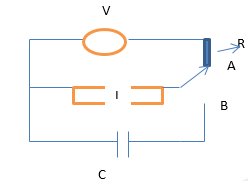Currently the switch is in position A and steady current is flowing to the circuit. Capacitor is uncharged. At t=0, switch is instanously moved to position B.
Question 13 Find the current in the LC circuit for t≥ 0
Here $\omega = \frac {1} {\sqrt LC}$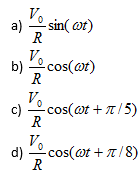Question 14 Find the charge q on the lower capacitor plate for t≥ 0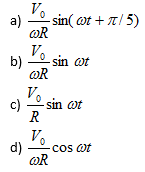Question 15 Which of the following is true of the circuit?
(a) The electric oscillations of time period $2\pi \sqrt{LC}$ are produced in the LC circuit
(b) The total energy remains conserved and is equal to $\frac {1}{2}L \frac {V_0^2}{R}$
(c) The maximum current in the circuit is V0/R
(d) None of these

Question 16
An LR circuit is connect to Voltage source V0. Which of the following is true at time equal to time constant
(a) The rate at which energy is being stored in Inductor is given by $\frac {.63 V_0^2}{R}$
(b) Power loss in the resistor is given by $\frac {.40 V_0^2}{R}$
(c) The rate of change of current is $\frac {.37 V_0^2}{R}$
(d) Current is 63% of the maximum value

Question 17
A circuit is shown below in Figure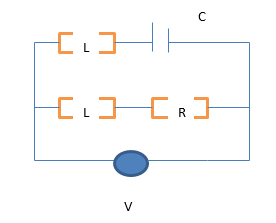It is given
L=R2C
Which of the following is true
(a) The total current will instantly rise to its final value V0/R when switch is closed
(b) The current will zero in LR branch and have value V0/R in the LC circuit path at t=0
(c) The current will rise with time in the LR circuit and decrease with time in LC circuit
(d) Total current in the circuit will always be V0/R for t>0

A sinusoidal voltage of frequency f=60Hz and peak value 150V is applied to a series LR circuit
Where L=40mH and R=20ohm
Question 18 Which one of the following is correct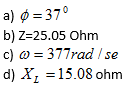Question 19 At t=T/6, which one of the following is true
(a) The instantaneous current is 2.344 A
(b) The instantaneous voltage across inductor is 83.29 V
(c) The instantaneous voltage across resistor is 46.88 V
(d) The total voltage applied is 130 V
Question 20 Which one of the following is true about the circuit?
(a) Average power into the circuit is 360 W
(b) Irms is 4.24 A
(c) Vrms  is  106.07 V
(d) None of these

Question 21:
A coil of inductance .50H and resistance 100 ohm is connected to 240 V,50Hz AC supply
Which of the following is true
a) Irms in the coil is 1.28 A
b) The time lag between voltage maximum and current maximum  is 3.2X10-3 s
c) Maximum Voltage is 339.36 V
d) None of these

Question 21
Consider a series RC circuit for which R = 2.0 × 106, C = 6.0 µF and E = 20 V. Find
(i) The time constant of the circuit,
(ii) The maximum charge on the capacitor after a switch in the circuit is closed, and
(iii) The current in the circuit at the instant just after the switch in the circuit is closed

A coil of resistance 15 Ohm and inductance .6 H is connected to a steady 120V power source.
The current starts from zero until it reaches it final state. $\frac{dI}{dt}$ is the rate of increase of current until it reaches steady state.

Question 22
Which equation will be correct until current reaches steady state
(a) $\frac{dI}{dt}=\frac{2-75I}{3}$
(b) $\frac{dI}{dt}=\frac{600-75I}{6}$
(c) $\frac{dI}{dt}=\frac{600-5I}{3}$
(d) $\frac{dI}{dt}=\frac{600-75I}{3}$

Question 23
Match the Following
Column A
(a) $\left(\frac{dI}{dt}\right)_{t=0}$
(b) $\left(\frac{dI}{dt}\right)$ when the current reaches 80% of the maximum values
(c) $\left(\frac{dI}{dt}\right)$ when the current reach the maximum value
(d) The current I when $\left(\frac{dI}{dt}\right)$ is 150 A/s

Column B
(p)0
(q)2
(r)40
(s) 200